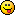<![CDATA[Math Is Fun Forum / Small Math Grammar Question]]> 2007-03-12T21:28:29Z FluxBB https://www.mathisfunforum.com/viewtopic.php?id=6355 <![CDATA[Re: Small Math Grammar Question]]> oh i was getting dazed

]]>
https://www.mathisfunforum.com/profile.php?id=6810 2007-03-12T21:28:29Z https://www.mathisfunforum.com/viewtopic.php?pid=63555#p63555
<![CDATA[Re: Small Math Grammar Question]]> Ok thanks all, what I was trying to explain was, that as F*D=F, F=/=0, then D = 1

"Or more generally, as the multiplied number doesn't equal zero and did not change, the number it was multiplied by must equal 1."

Or perhaps I should simply stick to using F and D instead of 'number' or 'term']]>
https://www.mathisfunforum.com/profile.php?id=5267 2007-03-10T13:03:08Z https://www.mathisfunforum.com/viewtopic.php?pid=63083#p63083
<![CDATA[Re: Small Math Grammar Question]]> If you think about it

So they can't all be terms, or you've got a pretty dire definition going on there.

]]>
https://www.mathisfunforum.com/profile.php?id=6243 2007-03-10T12:54:40Z https://www.mathisfunforum.com/viewtopic.php?pid=63082#p63082
<![CDATA[Re: Small Math Grammar Question]]> For y = mx+c, x and y are variables and m and c are constants. If you want to get really technical, then x is an independent variable and y is a dependant one (because you change x but y only changes depending on what x is).

If a, b and c can only take one value each then they are all constants. Unknown constants perhaps, but constants nonetheless.

I think your sentence makes sense, but I'm not sure because I don't know what context it's in. Also, if you're constructing some kind of proof then you'd better watch out for when the multiplied term was equal to 0, because then your statement isn't necessarily true.

]]>
https://www.mathisfunforum.com/profile.php?id=641 2007-03-10T12:52:59Z https://www.mathisfunforum.com/viewtopic.php?pid=63081#p63081
<![CDATA[Re: Small Math Grammar Question]]> Oh, so would the following passage be correct?

"Or more generally, as the multiplied term did not change, the term it was multiplied by must equal 1."

]]>
https://www.mathisfunforum.com/profile.php?id=5267 2007-03-10T12:29:24Z https://www.mathisfunforum.com/viewtopic.php?pid=63078#p63078
<![CDATA[Re: Small Math Grammar Question]]> I would say that a × b (or "ab") is a single term: Algebra - Definitions

]]>
https://www.mathisfunforum.com/profile.php?id=2 2007-03-10T12:07:06Z https://www.mathisfunforum.com/viewtopic.php?pid=63077#p63077
<![CDATA[Small Math Grammar Question]]> I know that if you have an equation...
a + b = c
...a, b, and c are terms.

However, if you have the equation...
a × b = c
...would you still be able to call a, b, and c terms? Would the product of a and b mean that they are the same term?

Also, are variables ANY pronumerals representing unknown values or are they only pronumerals which constantly change.
i.e. For y=mx+c, are all variables?
i.e. For a+b=c, where Each Letter Has 1 Possible Value, are any or all variables?

Thanks.

]]>
https://www.mathisfunforum.com/profile.php?id=5267 2007-03-10T12:03:03Z https://www.mathisfunforum.com/viewtopic.php?pid=63076#p63076